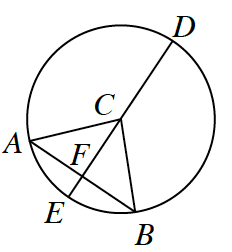### Home > INT2 > Chapter 8 > Lesson 8.4.1 > Problem8-85

8-85.A circle can be named by its center, as in circle $C$, or $\odot C$ at right. Larry started to set up a proof to show that if $\overline{AB} ⊥ \overline{DE}$ and $\overline{DE}$ is a diameter of $\odot C$, then $\overline{AF} ≅ \overline{FB}$. Examine his work below. Then complete his missing statements and reasons.

Statements

Reasons

1. $\overline{AB} ⊥ \overline{DE}$ and $\overline{DE}$ is a diameter of $\odot C$.

1. $∠AFC$ and $∠BFC$ are right angles.

1. $\overline{FC} ≅ \overline{FC}$

1. $\overline{AC} ≅ \overline{BC}$

1. Definition of a circle (radii must be equal)

1. $HL ≅$

1. $\overline{AF} ≅ \overline{FB}$

Click the check boxes as they appear in order to complete the proof.

1. Given

2. Definition of perpendicular

3. Reflexive Property

5. $ΔAFC≅ΔBFC$

6. $≅ Δ → ≅$ parts